# Class 8 RD Sharma Solutions – Chapter 17 Understanding Shapes Special Types Of Quadrilaterals – Exercise 17.2 | Set 1

• Last Updated : 21 Feb, 2021

### Question 1. Which of the following statements are true for a rhombus?

(i) It has two pairs of parallel sides.

(ii) It has two pairs of equal sides.

Attention reader! All those who say programming isn't for kids, just haven't met the right mentors yet. Join the  Demo Class for First Step to Coding Coursespecifically designed for students of class 8 to 12.

The students will get to learn more about the world of programming in these free classes which will definitely help them in making a wise career choice in the future.

(iii) It has only two pairs of equal sides.

(iv) Two of its angles are at right angles.

(v) Its diagonals bisect each other at right angles.

(vi) Its diagonals are equal and perpendicular.

(vii) It has all its sides of equal lengths.

(viii) It is a parallelogram.

(x) It can be a square.

(xi) It is a square.

Solution:

(i) True

(ii) True

(iii) False. A rhombus has all four sides equal.

(iv) False. A rhombus has no right angle.

(v) True

(vi) False. Rhombus diagonals are unequal in length.

(vii) True

(viii) True

(ix) True

(x) True. Rhombus becomes square when any one angle is 90°.

(xi) False. Rhombus is never a square. Since in a square each angle is 90°.

### Question 2. Fill in the blanks, in each of the following, so as to make the statement true:

(i) A rhombus is a parallelogram in which _______.

(ii) A square is a rhombus in which _________.

(iii) A rhombus has all its sides of ______ length.

(iv) The diagonals of a rhombus _____ each other at ______ angles.

(v) If the diagonals of a parallelogram bisect each other at right angles, then it is a ______.

Solution:

(ii) any one angle is right angle.

(iii) equal

(iv) bisect, right

(v) rhombus

### Question 3. The diagonals of a parallelogram are not perpendicular. Is it a rhombus? Why or why not?

Solution:

No, Diagonals of a rhombus bisect each other at 90°, where the diagonals must be perpendicular to each other.

Solution:

No, since the diagonals of a rhombus are perpendicular to each other and bisect. Also, its sides are equal.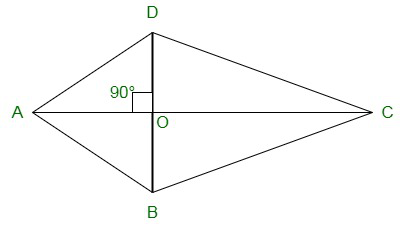### Question 5. ABCD is a rhombus. If ∠ACB = 40°, find ∠ADB.

Solution: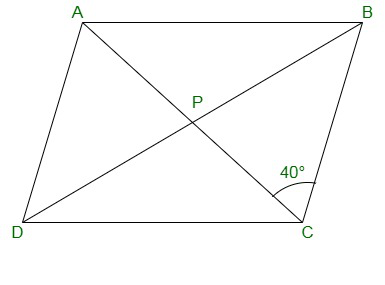ABCD is a rhombus where the diagonals are perpendicular to each other.

So, ∠BOC = 90o

From ΔBOC, the sum of angles in a triangle is 180o

Therefore,

∠CBO + ∠BOC + ∠OBC = 180o

Solving, we get,

= 180o – 40o – 90o

= 50o

Since, the alternate angles are equal,

∴ ∠ADB = ∠CBO = 50o

### Question 6. If the diagonals of a rhombus are 12 cm and 16 cm, find the length of each side.

Solution: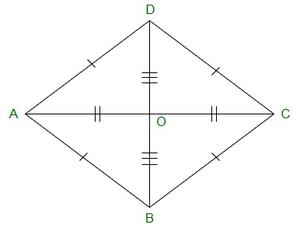In a rhombus, diagonals bisect each other at right angle.

Now, In ΔAOB

AO = 12/2 = 6cm, BO = 16/2 = 8cm

AB2 = AO2 + BO2 (Pythagoras Theorem)

AB2 = 62 + 82

AB2 = 36 + 64

AB2 = 100

AB =√100 = 10cm

Therefore, each side of a rhombus is 10cm.

### Question 7. Construct a rhombus whose diagonals are of length 10 cm and 6 cm.

Solution: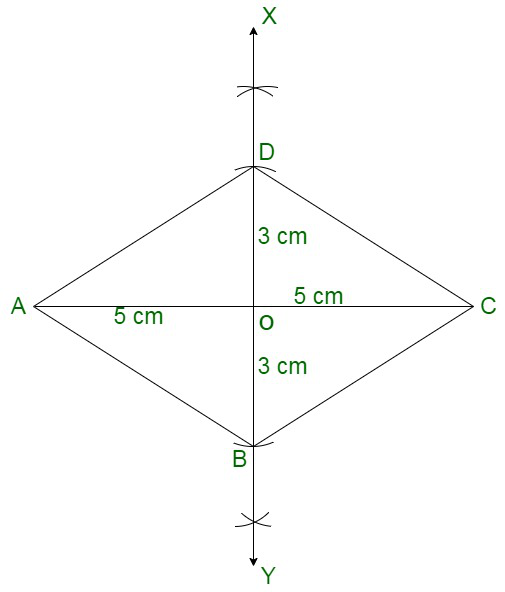Steps to Construct a rhombus:

(i) Draw a diagonal AC of length 10 cm.

(ii) Taking O as centre, draw a perpendicular bisector of AC to point O.

(iii) From point ‘O’ cut two arcs of length 3cm each to get points OB and OD.

(iv) Join the line segments AD and AB, BC and CD to get rhombus ABCD.

### Question 8. Draw a rhombus, having each side of length 3.5 cm and one of the angles as 40°.

Solution: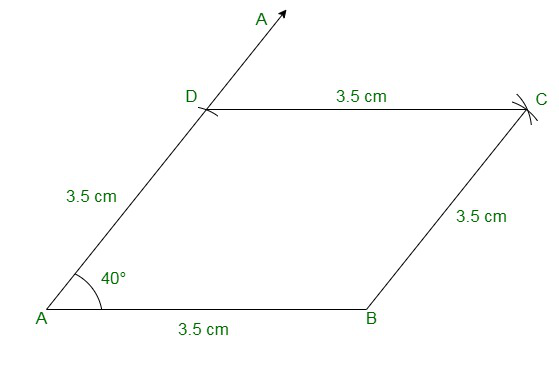Steps to construct a rhombus:

(i) Draw a line segment AB of length 3.5 cm.

(ii) From point A and B construct two angles of 40 and 140 degrees respectively.

(iii) From points A and B cut two arcs of length 3.5 cm each to get the points D and C.

(iv) Join the line segments AD, BC, CD to get rhombus ABCD.

My Personal Notes arrow_drop_up